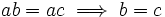# Difference between revisions of "Varying group"

This is a survey article related to:group
View other survey articles about group

In this article, we explore many variations that are possible in the definition of a group, that yield similar, though slightly weaker or stronger, structures.

To begin with, let us recall the three axioms that describe a group:

• The associativity of the binary operation
• The existence of a neutral element (viz, a left and right multiplicative identity)
• The existence of a multiplicative inverse for every element

The idea behind varying the notion of group would be to remove, or relax, one or more of these assumptions.

## Relaxing the inverse element assumption

### Group without inverses: Monoid

Further information: monoid

A set equipped with an associative binary operation having a neutral element (viz, left and right multiplicative identity) is termed a monoid. Monoids differ from groups in the sense of not necessarily having inverses. Examples of monoids are the set of natural numbers under multiplication, the set of integers under multiplication, the set of integers modulo$n$ under multiplication, the set of all functions from a set to itself (under composition), and more generally, the set of all endomorphisms of a structure.

### Cancellation monoid

Further information: Cancellation monoid

Due to the existence of inverses in a group we can do left and right cancellation. Let's run through the proof quickly:$ab = ac \implies a^{-1}(ab) = a^{-1}(ac) \implies (a^{-1}a)b = (a^{-1}a)c \implies b = c$

A natural question: are there monoids where we can do both left and right cancellation, viz:$ab = ac \implies b = c$

and$ac = bc \implies a = b$

even without assuming the existence of inverses? Actually there do exist such monoids, and such monoids are called cancellation monoids. The following are true:

• Any submonoid of a cancellation monoid is a cancellation monoid. In particular, any submonoid of a group is a cancellation monoid. Thus, the natural numbers under multiplication form a cancellation monoid, because they can be embedded in the group of nonzero rationals under multiplication.
• Any Abelian cancellation monoid can be embedded as a submonoid of an Abelian group. However, the corresponding statement is not true for general cancellation monoids: there are cancellation monoids that cannot be embedded into any group.

## Relaxing the neutral element assumption

At first sight, it seems hard to have a notion of inverse without having a corresponding notion of identity element. However, there are clever ways of doing this, as we see below.

### Without neutral element or inverse

Further information: Semigroup

A set equipped with an associative binary operation, without any assumption of neutral element or inverses, is termed a semigroup.

### Inverse elements without reference to neutral element

For a group, we define two elements$a$ and$b$ to be inverse if$ab = ba = e$. This definition of inverse element involves the use of neutral element. However, a more general notion of inverse would be of two elements which reverse the action of each other. In other words, doing$a$, then$b$ may not land you right where you were to begin with, but doing$a$ again should have the same effect as doing$a$ once. In other words, we want:$a = aba$ and$b = bab$.

This gives rise to notions of regular element and inverse element. A semigroup where every element is regular (respectively inverse) is termed a regular semigroup (respectively inverse semigroup).

## Relaxing the associativity assumption

Of all the assumptions, associativity is the most common, and in some sense, the most basic assumption. Thus, relaxing, or removing, the associativity assumption, is a drastic step.

### Set with only a binary operation

Further information: Magma

A set equipped with a binary operation (with no assumptions of asociativity, neutral element, or inverses) is termed a magma. In the literature, this is sometimes also referred to as a groupoid but the term groupoid also has other meanings.

### The accessibility relation and quasigroups

Further information: Quasigroup

We can define the following relation on any magma. We say$b$ is left-accessible from$a$ if there is a$x$ such that$x * a = b$. Now, if the magma were associative, then left-accessibility would be a transitive relation. In general, we cannot say that about left-accessibility.

Similarly, we can define a relation of being right-accessible. Again, if the magma is associative, then right-accessibility is a transitive relation. In general, we cannot say that about right-accessibility.

Groups have a powerful property: every element is left-accessible, as well as right-accessible, from every other. This is a consequence of the existence of inverses. This suggests an analogous definition in the non-associative case.

A quasigroup is a magma with the property that given any$a$ and$b$ there are unique elements$x$ and$y$ such that$x * a = a * y = b$. Equivalently, a quasigroup is a magma where:

• Every element is left-accessible, and right-accessible, from every other
• We can always do both left and right cancellation

Every associative quasigroup is a group.

Also, finite quasigroups are in bijection with Latin squares.

### Algebra loops

Further information: Algebra loop

An algebra loop is a quasigroup which possesses a neutral element (for multiplication).

### Power-associativity

Further information: Power-associative binary operation

A binary operation is said to be power-associative if all powers of an element associate, and hence for any natural number$n$, all parenthesizations of$x^n$ are equivalent. We can study quasigroups where power-associativity holds.

### Alternativity

Further information: Alternative binary operation

A magma is said to be alternative if the submagma generated by any two elements is associative. We are often interested in alternative algebra loops.

## Variations at the group algebra level

Some of the approaches we use to vary groups do not work at the level of group, but rather, require us to perform variation on the group algebra.

Further information: Varying the group algebra